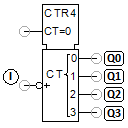# 4-bit counterThe counter counts the number of falling edges from 1 to 0 at input I. The number is saved as a 4-bit number x and output bit-by-bit via the outputs Q0, Q1, Q2 and Q3. The counter is reset to x=0 after the value x=15 is reached. The counter can also be set to 0 with the value 1 at the input CT=0. The counter x is output to the outputs as follows:

x = Q0*2^0 + Q1*2^1 + Q2*2^2 + Q3*2^3

The IC 74HC93 is used as a component in the training package TP 1012 from Festo Didactic.